Paparazzi UAS  v5.17_devel-14-g4575375 Paparazzi is a free software Unmanned Aircraft System.
air_data.h File Reference

Air Data interface. More...

#include "std.h"Include dependency graph for air_data.h:This graph shows which files directly or indirectly include this file:

Go to the source code of this file.

## Data Structures

struct  AirData
Air Data strucute. More...

## Functions

void air_data_init (void)
AirData initialization. More...

void air_data_periodic (void)
Check health. More...

float air_data_get_amsl (void)
Return AMSL (altitude AboveSeaLevel). More...

float eas_from_dynamic_pressure (float q)
Calculate equivalent airspeed from dynamic pressure. More...

float get_tas_factor (float p, float t)
Calculate true airspeed (TAS) factor. More...

float tas_from_eas (float eas)
Calculate true airspeed from equivalent airspeed. More...

float tas_from_dynamic_pressure (float q)
Calculate true airspeed from dynamic pressure. More...

## Variables

struct AirData air_data
global AirData state More...

## Detailed Description

Air Data interface.

• pressures
• airspeed
• angle of attack and sideslip
• wind

Definition in file air_data.h.

## Data Structure Documentation

 struct AirData

Air Data strucute.

Definition at line 37 of file air_data.h.

Data Fields
float airspeed Equivalent Air Speed (equals to Calibrated Air Speed at low speed/altitude) (in m/s, -1 if unknown.
float amsl_baro altitude above sea level in m from pressure and QNH
bool amsl_baro_valid TRUE if amsl_baro is currently valid.
float aoa angle of attack (rad)
bool calc_airspeed if TRUE, calculate airspeed from differential pressure
bool calc_amsl_baro if TRUE, calculate amsl_baro
bool calc_qnh_once flag to calculate QNH with next pressure measurement
bool calc_tas_factor if TRUE, calculate tas_factor when getting a temp measurement
float differential Differential pressure (total - static pressure) (Pa)
float pressure Static atmospheric pressure (Pa), -1 if unknown.
float qnh Barometric pressure adjusted to sea level in hPa, -1 if unknown.
float tas True Air Speed (TAS) in m/s, -1 if unknown.
float tas_factor factor to convert equivalent airspeed (EAS) to true airspeed (TAS)
float temperature temperature in degrees Celcius, -1000 if unknown
float wind_dir wind direction (rad, 0 north, >0 clockwise)
float wind_speed wind speed (m/s)

## Function Documentation

 float air_data_get_amsl ( void )

Return AMSL (altitude AboveSeaLevel).

If AMSL from baro is valid, return that, otherwise from gps.

Definition at line 257 of file air_data.c.

Referenced by tas_from_eas().Here is the call graph for this function:Here is the caller graph for this function:

 void air_data_init ( void )

AirData initialization.

Called at startup.

Called at startup. Bind ABI messages

Definition at line 216 of file air_data.c.Here is the call graph for this function:

 void air_data_periodic ( void )

Check health.

Needs to be called periodically.

Definition at line 267 of file air_data.c.

References air_data, and AirData::amsl_baro_valid.

 float eas_from_dynamic_pressure ( float q )

Calculate equivalent airspeed from dynamic pressure.

Dynamic pressure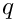(also called impact pressure) is the difference between total(pitot) and static pressure.

Parameters
 q dynamic pressure in Pa
Returns
equivalent airspeed in m/s

Dynamic pressure(also called impact pressure) is the difference between total(pitot) and static pressure.

Airspeed from dynamic pressure: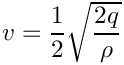with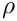as air density. Using standard sea level air density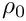gives you equivalent airspeed (EAS).

Parameters
 q dynamic pressure in Pa
Returns
equivalent airspeed in m/s

Definition at line 291 of file air_data.c.

References Max, and PPRZ_ISA_AIR_DENSITY.

Referenced by pressure_diff_cb(), and tas_from_dynamic_pressure().Here is the caller graph for this function:

 float get_tas_factor ( float p, float t )

Calculate true airspeed (TAS) factor.

TAS = tas_factor * EAS

Parameters
 p current air pressure in Pa t current air temperature in degrees Celcius
Returns
tas factor

TAS = tas_factor * EAS

True airspeed (TAS) from equivalent airspeed (EAS):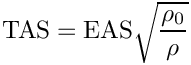and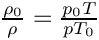where

•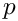is the air pressure at the flight condition
•is the air pressure at sea level = 101325 Pa
•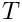is the air temperature at the flight condition
•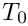is the air temperature at sea level = 288.15 K
Parameters
 p current air pressure in Pa t current air temperature in degrees Celcius
Returns
tas factor

Definition at line 319 of file air_data.c.

References KelvinOfCelsius, PPRZ_ISA_SEA_LEVEL_PRESSURE, and PPRZ_ISA_SEA_LEVEL_TEMP.

Referenced by tas_from_eas(), and temperature_cb().Here is the caller graph for this function:

 float tas_from_dynamic_pressure ( float q )

Calculate true airspeed from dynamic pressure.

Dynamic pressure(also called impact pressure) is the difference between total(pitot) and static pressure.

Parameters
 q dynamic pressure in Pa
Returns
true airspeed in m/s

Definition at line 365 of file air_data.c.

References eas_from_dynamic_pressure(), and tas_from_eas().

Referenced by pressure_diff_cb().Here is the call graph for this function:Here is the caller graph for this function:

 float tas_from_eas ( float eas )

Calculate true airspeed from equivalent airspeed.

Parameters
 eas equivalent airspeed (EAS) in m/s
Returns
true airspeed in m/s

True airspeed (TAS) from EAS: TAS = air_data.tas_factor * EAS

Parameters
 eas equivalent airspeed (EAS) in m/s
Returns
true airspeed in m/s

Definition at line 338 of file air_data.c.

Referenced by airspeed_cb(), pressure_diff_cb(), and tas_from_dynamic_pressure().Here is the call graph for this function:Here is the caller graph for this function:

## Variable Documentation

 struct AirData air_data

global AirData state

Definition at line 39 of file air_data.c.